# RD Sharma Solutions Class 12 Linear Programming Exercise 30.1

RD Sharma Solutions for Class 12 Maths Chapter 30 Linear Programming Exercise 30.1, is provided here to help students come out with flying colours in their board examinations. Our expert faculty team have solved the questions in a step by step format, which helps students understand the concepts clearly. Start practising offline by downloading the pdf of RD Sharma Solutions for Class 12 now and these can be used for future reference, as well.

## Download PDF of RD Sharma Solutions for Class 12 Maths Chapter 30 Exercise 1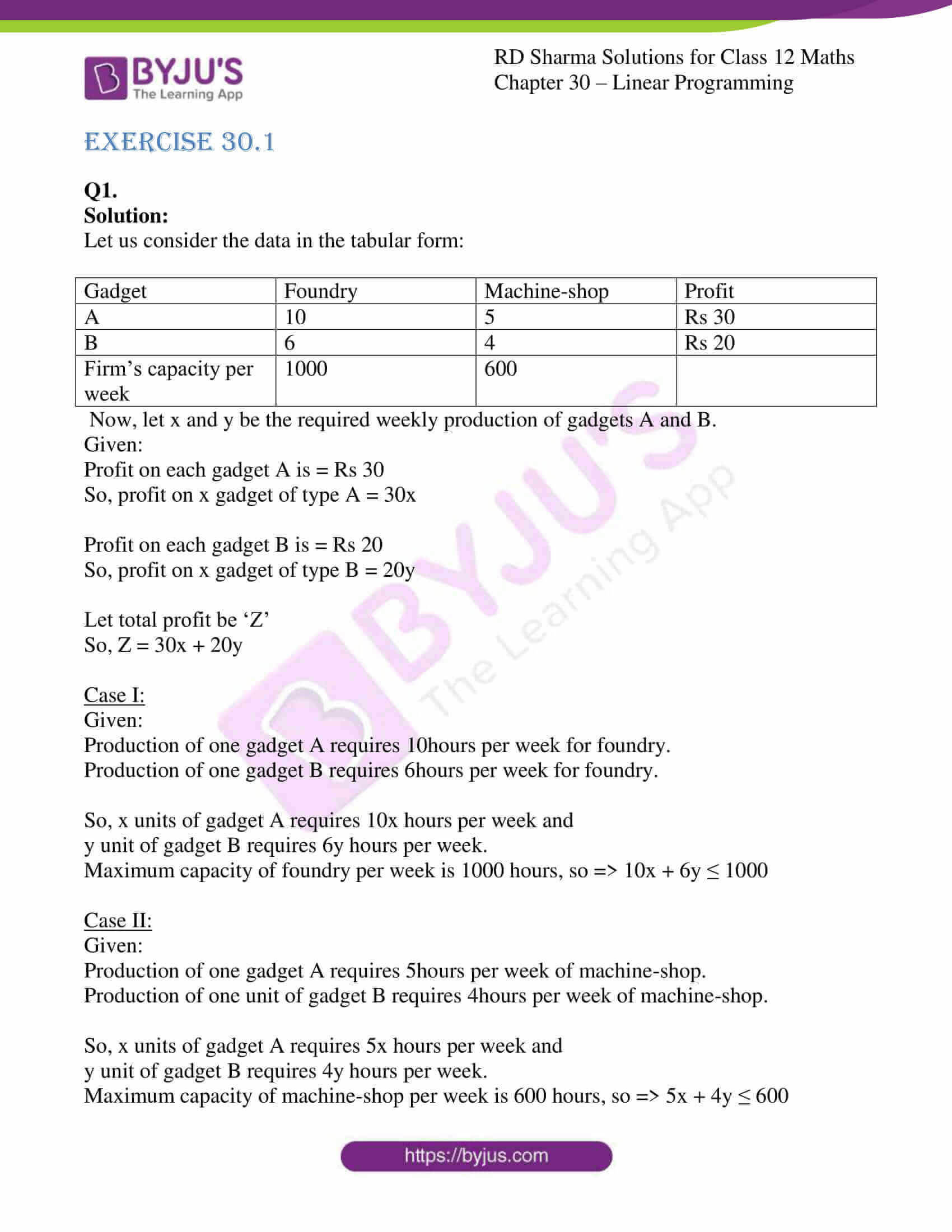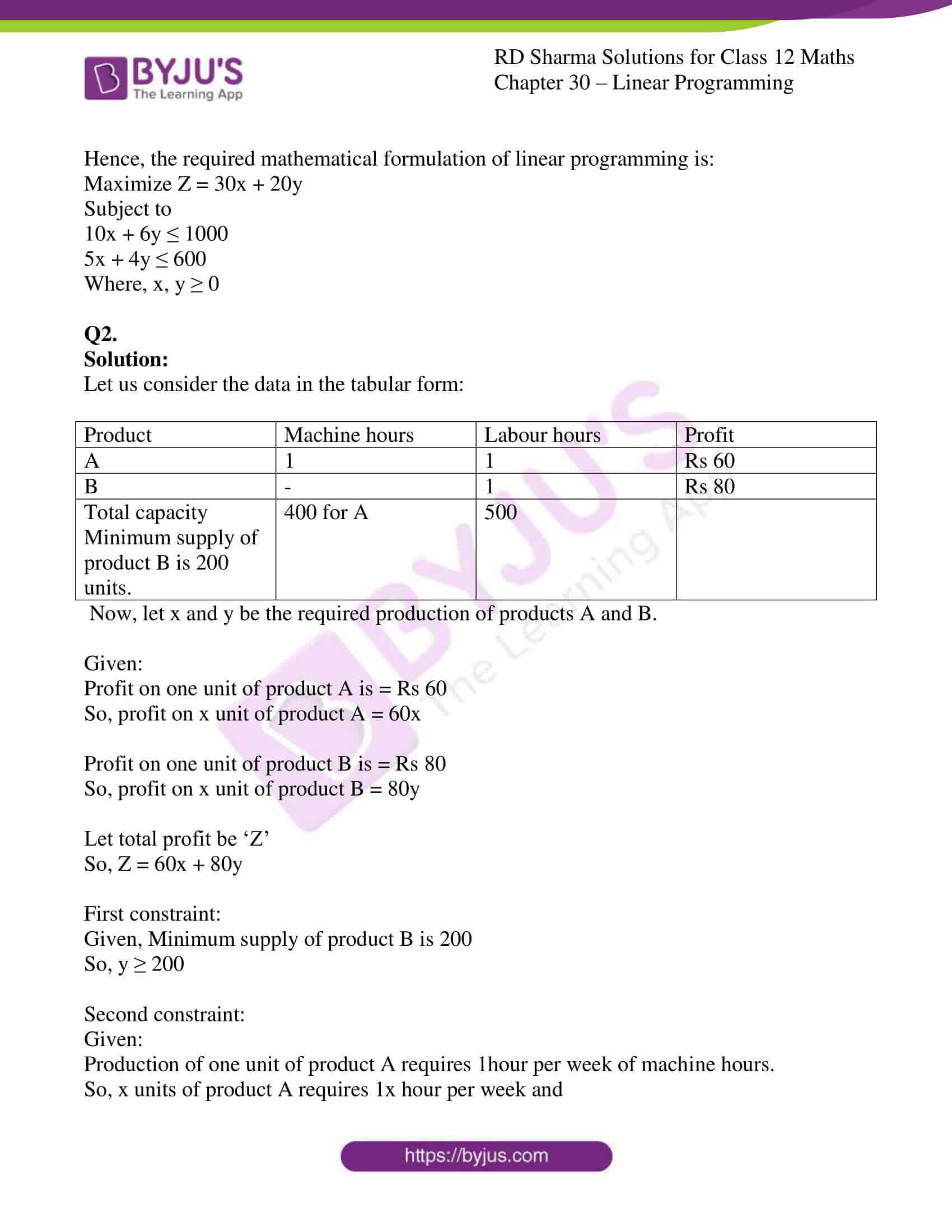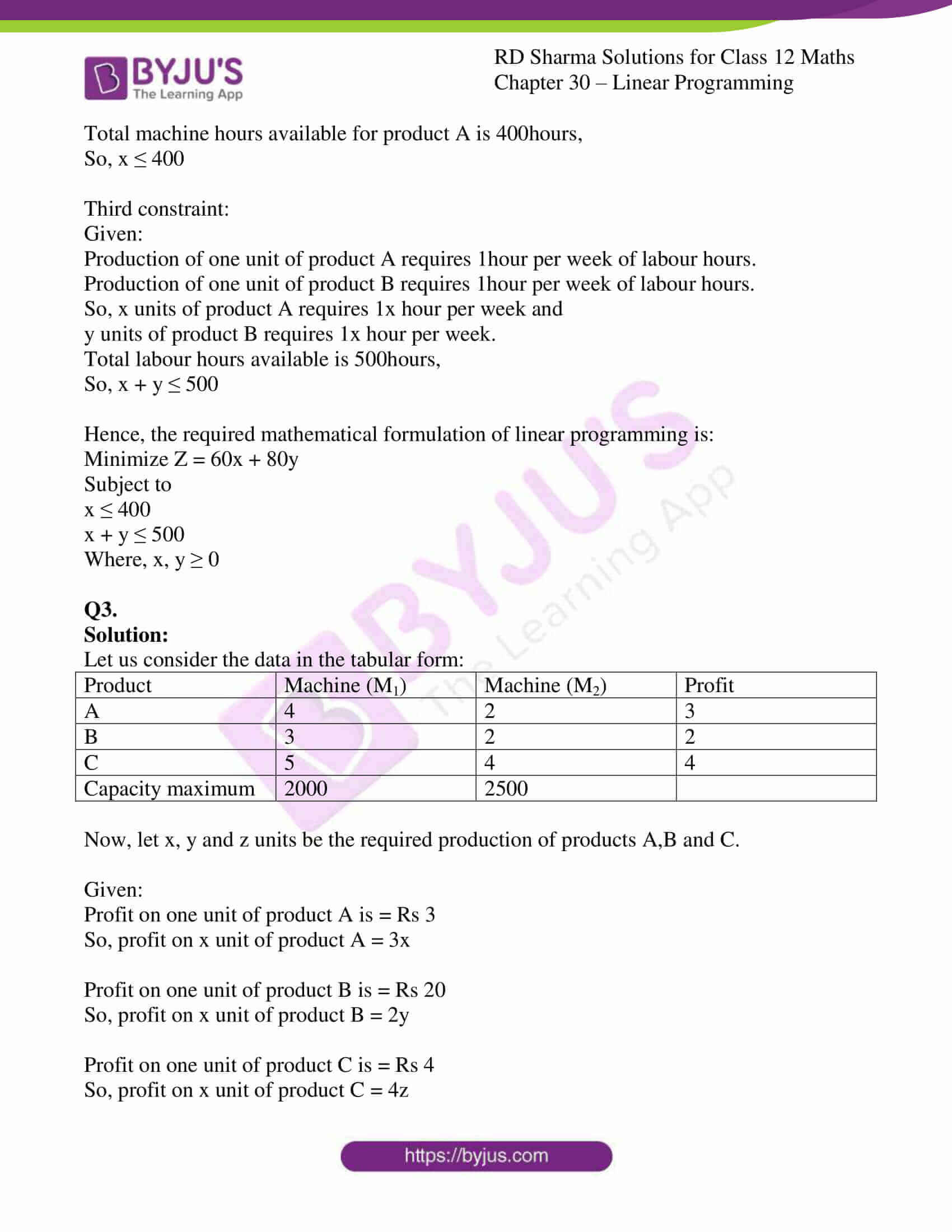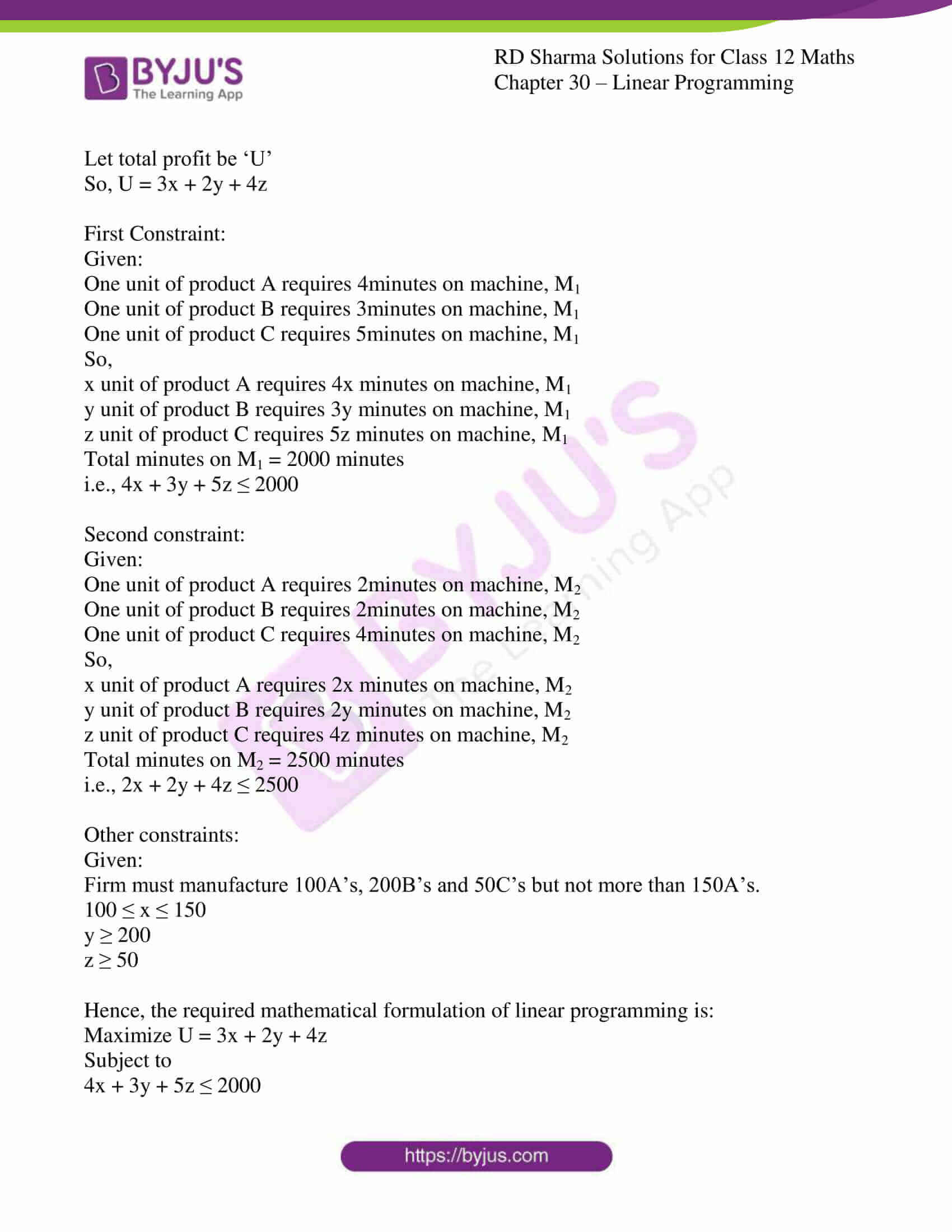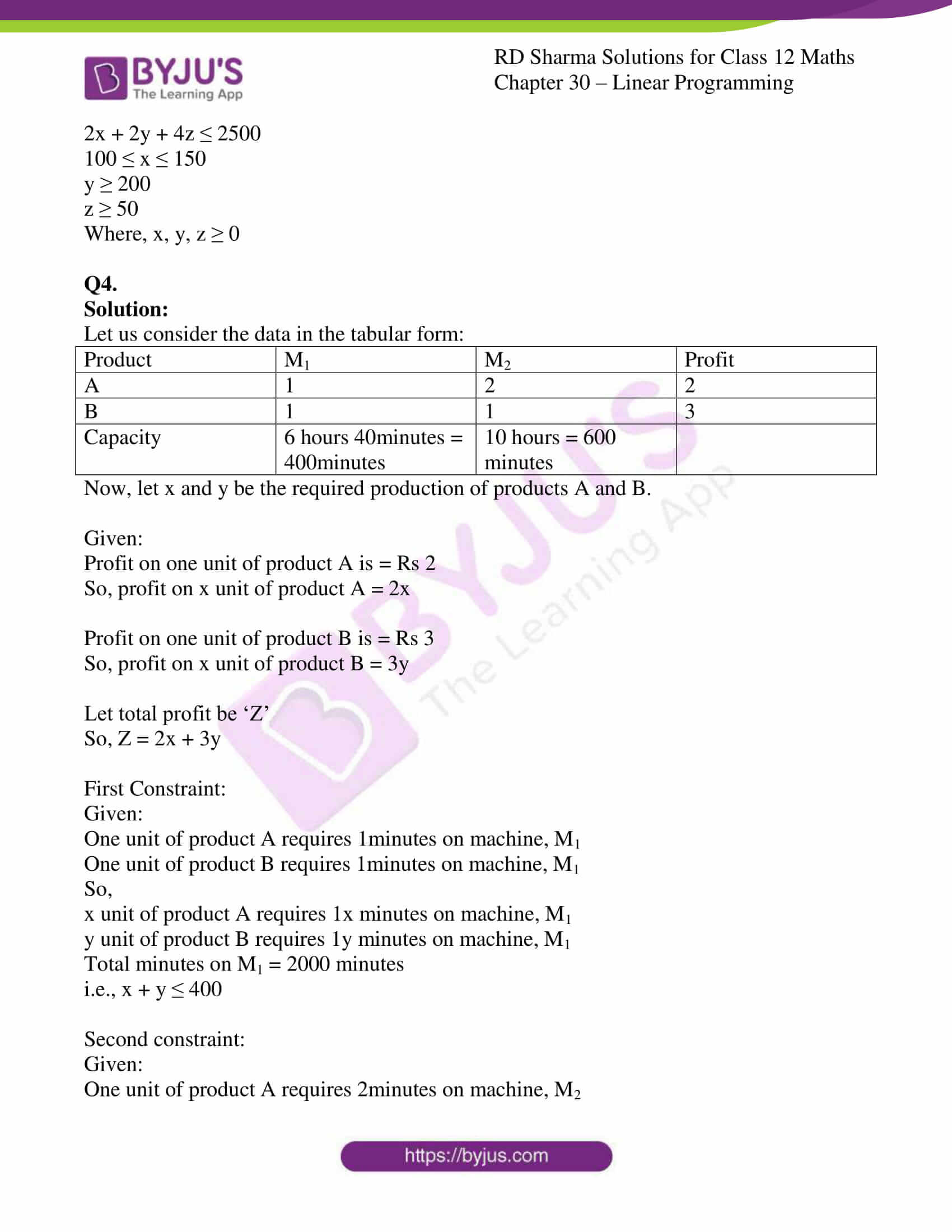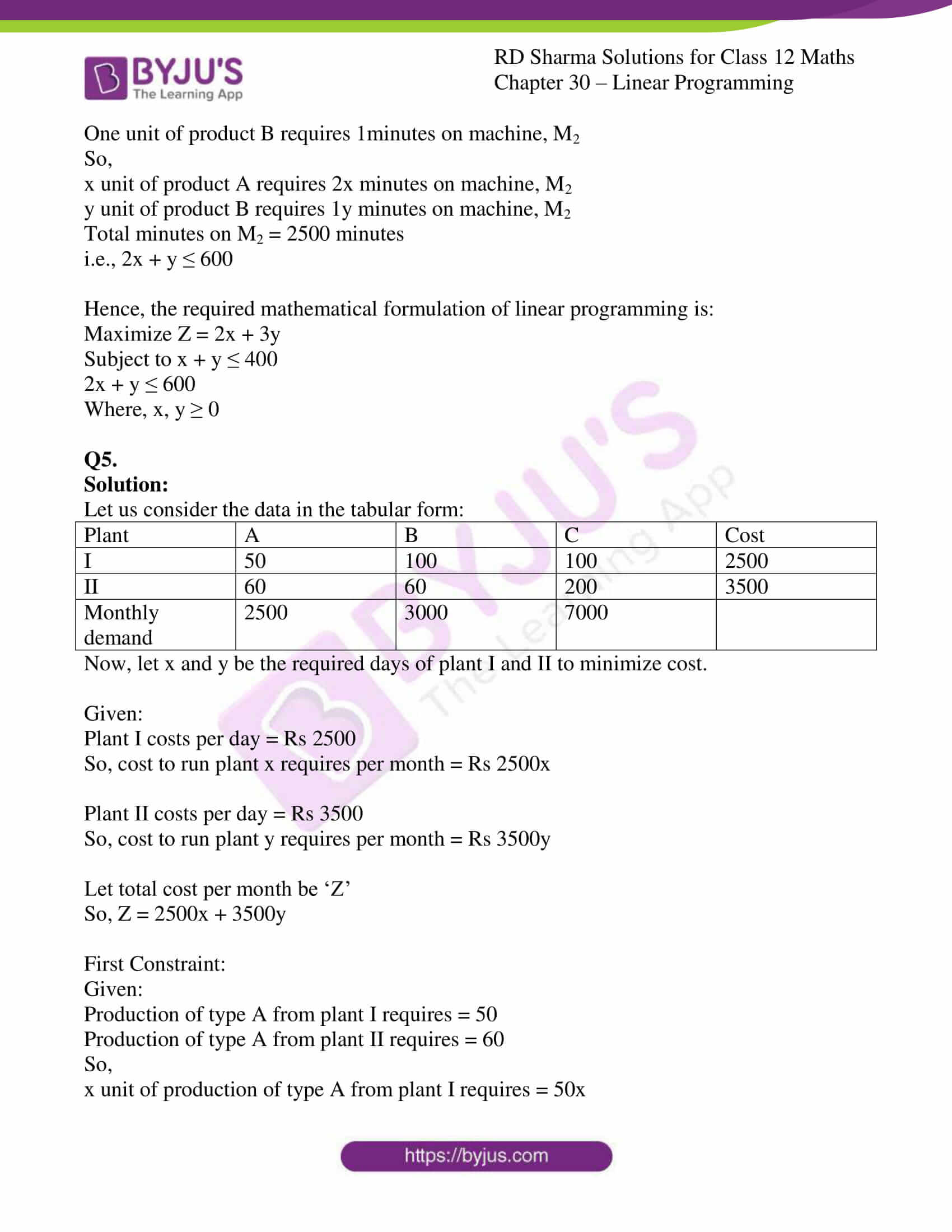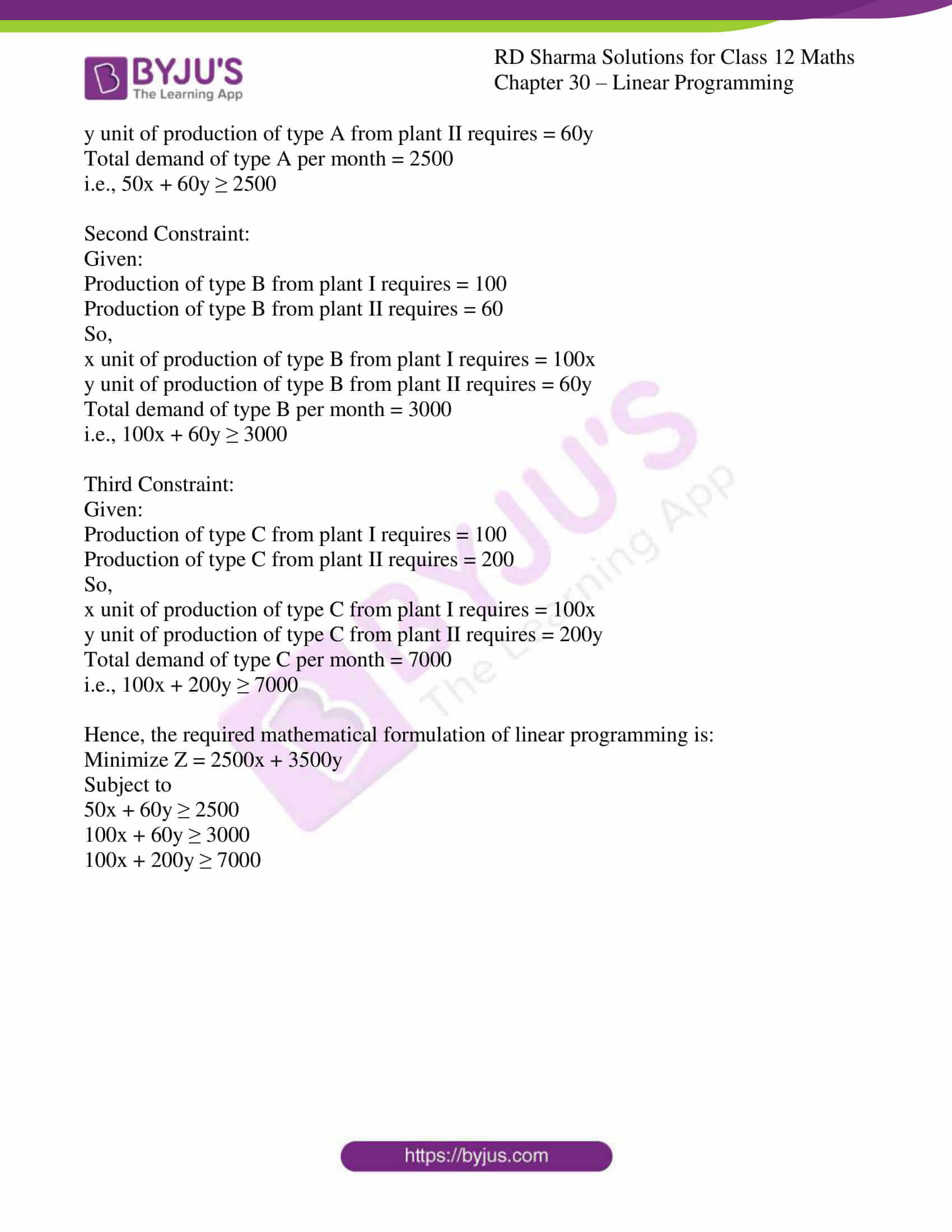### Access RD Sharma Solutions for Class 12 Maths Chapter 30 Exercise 1

EXERCISE 30.1

Q1.

Solution:

Let us consider the data in the tabular form:

 Gadget Foundry Machine-shop Profit A 10 5 Rs 30 B 6 4 Rs 20 Firm’s capacity per week 1000 600

Now, let x and y be the required weekly production of gadgets A and B.

Given:

Profit on each gadget A is = Rs 30

So, profit on x gadget of type A = 30x

Profit on each gadget B is = Rs 20

So, profit on x gadget of type B = 20y

Let total profit be ‘Z’

So, Z = 30x + 20y

Case I:

Given:

Production of one gadget A requires 10hours per week for foundry.

Production of one gadget B requires 6hours per week for foundry.

So, x units of gadget A requires 10x hours per week and

y unit of gadget B requires 6y hours per week.

Maximum capacity of foundry per week is 1000 hours, so => 10x + 6y ≤ 1000

Case II:

Given:

Production of one gadget A requires 5hours per week of machine-shop.

Production of one unit of gadget B requires 4hours per week of machine-shop.

So, x units of gadget A requires 5x hours per week and

y unit of gadget B requires 4y hours per week.

Maximum capacity of machine-shop per week is 600 hours, so => 5x + 4y ≤ 600

Hence, the required mathematical formulation of linear programming is:

Maximize Z = 30x + 20y

Subject to

10x + 6y ≤ 1000

5x + 4y ≤ 600

Where, x, y ≥ 0

Q2.

Solution:

Let us consider the data in the tabular form:

 Product Machine hours Labour hours Profit A 1 1 Rs 60 B – 1 Rs 80 Total capacity Minimum supply of product B is 200 units. 400 for A 500

Now, let x and y be the required production of products A and B.

Given:

Profit on one unit of product A is = Rs 60

So, profit on x unit of product A = 60x

Profit on one unit of product B is = Rs 80

So, profit on x unit of product B = 80y

Let total profit be ‘Z’

So, Z = 60x + 80y

First constraint:

Given, Minimum supply of product B is 200

So, y ≥ 200

Second constraint:

Given:

Production of one unit of product A requires 1hour per week of machine hours.

So, x units of product A requires 1x hour per week and

Total machine hours available for product A is 400hours,

So, x ≤ 400

Third constraint:

Given:

Production of one unit of product A requires 1hour per week of labour hours.

Production of one unit of product B requires 1hour per week of labour hours.

So, x units of product A requires 1x hour per week and

y units of product B requires 1x hour per week.

Total labour hours available is 500hours,

So, x + y ≤ 500

Hence, the required mathematical formulation of linear programming is:

Minimize Z = 60x + 80y

Subject to

x ≤ 400

x + y ≤ 500

Where, x, y ≥ 0

Q3.

Solution:

Let us consider the data in the tabular form:

 Product Machine (M1) Machine (M2) Profit A 4 2 3 B 3 2 2 C 5 4 4 Capacity maximum 2000 2500

Now, let x, y and z units be the required production of products A,B and C.

Given:

Profit on one unit of product A is = Rs 3

So, profit on x unit of product A = 3x

Profit on one unit of product B is = Rs 20

So, profit on x unit of product B = 2y

Profit on one unit of product C is = Rs 4

So, profit on x unit of product C = 4z

Let total profit be ‘U’

So, U = 3x + 2y + 4z

First Constraint:

Given:

One unit of product A requires 4minutes on machine, M1

One unit of product B requires 3minutes on machine, M1

One unit of product C requires 5minutes on machine, M1

So,

x unit of product A requires 4x minutes on machine, M1

y unit of product B requires 3y minutes on machine, M1

z unit of product C requires 5z minutes on machine, M1

Total minutes on M1 = 2000 minutes

i.e., 4x + 3y + 5z ≤ 2000

Second constraint:

Given:

One unit of product A requires 2minutes on machine, M2

One unit of product B requires 2minutes on machine, M2

One unit of product C requires 4minutes on machine, M2

So,

x unit of product A requires 2x minutes on machine, M2

y unit of product B requires 2y minutes on machine, M2

z unit of product C requires 4z minutes on machine, M2

Total minutes on M2 = 2500 minutes

i.e., 2x + 2y + 4z ≤ 2500

Other constraints:

Given:

Firm must manufacture 100A’s, 200B’s and 50C’s but not more than 150A’s.

100 ≤ x ≤ 150

y ≥ 200

z ≥ 50

Hence, the required mathematical formulation of linear programming is:

Maximize U = 3x + 2y + 4z

Subject to

4x + 3y + 5z ≤ 2000

2x + 2y + 4z ≤ 2500

100 ≤ x ≤ 150

y ≥ 200

z ≥ 50

Where, x, y, z ≥ 0

Q4.

Solution:

Let us consider the data in the tabular form:

 Product M1 M2 Profit A 1 2 2 B 1 1 3 Capacity 6 hours 40minutes = 400minutes 10 hours = 600 minutes

Now, let x and y be the required production of products A and B.

Given:

Profit on one unit of product A is = Rs 2

So, profit on x unit of product A = 2x

Profit on one unit of product B is = Rs 3

So, profit on x unit of product B = 3y

Let total profit be ‘Z’

So, Z = 2x + 3y

First Constraint:

Given:

One unit of product A requires 1minutes on machine, M1

One unit of product B requires 1minutes on machine, M1

So,

x unit of product A requires 1x minutes on machine, M1

y unit of product B requires 1y minutes on machine, M1

Total minutes on M1 = 2000 minutes

i.e., x + y ≤ 400

Second constraint:

Given:

One unit of product A requires 2minutes on machine, M2

One unit of product B requires 1minutes on machine, M2

So,

x unit of product A requires 2x minutes on machine, M2

y unit of product B requires 1y minutes on machine, M2

Total minutes on M2 = 2500 minutes

i.e., 2x + y ≤ 600

Hence, the required mathematical formulation of linear programming is:

Maximize Z = 2x + 3y

Subject to x + y ≤ 400

2x + y ≤ 600

Where, x, y ≥ 0

Q5.

Solution:

Let us consider the data in the tabular form:

 Plant A B C Cost I 50 100 100 2500 II 60 60 200 3500 Monthly demand 2500 3000 7000

Now, let x and y be the required days of plant I and II to minimize cost.

Given:

Plant I costs per day = Rs 2500

So, cost to run plant x requires per month = Rs 2500x

Plant II costs per day = Rs 3500

So, cost to run plant y requires per month = Rs 3500y

Let total cost per month be ‘Z’

So, Z = 2500x + 3500y

First Constraint:

Given:

Production of type A from plant I requires = 50

Production of type A from plant II requires = 60

So,

x unit of production of type A from plant I requires = 50x

y unit of production of type A from plant II requires = 60y

Total demand of type A per month = 2500

i.e., 50x + 60y ≥ 2500

Second Constraint:

Given:

Production of type B from plant I requires = 100

Production of type B from plant II requires = 60

So,

x unit of production of type B from plant I requires = 100x

y unit of production of type B from plant II requires = 60y

Total demand of type B per month = 3000

i.e., 100x + 60y ≥ 3000

Third Constraint:

Given:

Production of type C from plant I requires = 100

Production of type C from plant II requires = 200

So,

x unit of production of type C from plant I requires = 100x

y unit of production of type C from plant II requires = 200y

Total demand of type C per month = 7000

i.e., 100x + 200y ≥ 7000

Hence, the required mathematical formulation of linear programming is:

Minimize Z = 2500x + 3500y

Subject to

50x + 60y ≥ 2500

100x + 60y ≥ 3000

100x + 200y ≥ 7000